Deutsche VersionConverting Decibel to Percentage (%) and vice versa

● Conversion of Distortion to Decibel ●

Signal distortionTotal Harmonic Distortion

Conversion of THD to dB and percentage and vice versa
Distortion attenuation a in dB ← → Distortion factor k in %

 Nonlinear distortions are given as distortion attenuation ak in dB or as damping factor k (THD) in percent. Total harmonic distortion (THD) is defined as the ratio of the rms voltage of the harmonics to that of the fundamental component. This is accomplished by using a spectrum analyzer to obtain the level of each harmonic and performing an rms summation. The level is divided by the fundamental level, and cited as the total harmonic distortion (expressed in percent). Also the expression THD = Total Harmonic Distortion for signal amplitude distortion is well known. The English expression THD = Total Harmonic Distortion is usual for the size distortion Total harmonic distortion. This is the level difference between Harmonic Distortion (unwanted overtones) and the total signal in dB; see distortion. The distortion of an audio device indicates the extent to which a sinusoidal input signal (test tone amplitude) by non-linear distortions unwanted overtones and harmonics are added. It is therefore a measure of the occurring harmonic distortion. The value is given in percent, relative to the total signal. Harmonic distortion can be found with a minus sign in front of the dB value, the harmonic distortion is also specified with a positive sign. There are variables that have no unit. These include ratios that are often connected with the improper unit percent (%) or decibel (dB).

Conversion of distortion attenuation ak as damping in decibel (dB)
to distortion factor
k (THD) as percent value %

 Distortion attenuation a dB | Distortion factor THD (k) % | ↓ | ↓ | Distortion factor THD (k) % | Distortion attenuation a dB |
 Fill out the gray box and click at the respective calculation bar under it. Don't forget to enter the minus sign for the distortion attenuation in decibels.

 The distortion factor is always less than 1 or less than 100%, therefore, the total harmonic distortion has to be always be a negative dB value. Total harmonic distortion:in dB. Input k in percent. Total harmonic distortion (THD)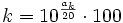in %. Input ak in dB with a minus sign. To calculate the distortion factor, there are two different formulas: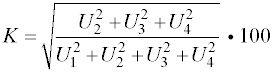= Distortion att. up to 5%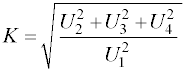× 100 = THD U = voltage. The distortion factor was historically introduced before the THD-value and the ratio of the effective value of all harmonics to the RMS value of all harmonics plus fundamental frequency, that is the total signal. The THD is the ratio of RMS signal of all harmonics to the RMS of the fundamental signal.   "THD expressed as a power ratio" is really not used in sound recording techniques. We use amplitude or voltage.   It is also common to specify the THD + N, where N stands for noise. THD+N. This test measures everything added to the input signal. Great, since everything that comes out of the unit that isn't the pure test signal is measured and included − harmonics, hum, noise, RFI, buzz ... everything.

 The distortion factor is always smaller than 1 or less than 100 %, therefore the distortion attenuation has to be a negative dB value. Distortion attenuation:in dB. Input k in percent. Distortion factor THD:in %. Input ak in dB with a minus sign.

Table: Distortions and the dB
and percent and the decibel

Distortions as voltage or sound pressure belong to field quantities

 THD k k (%) ak (dB) factor percent decibel 1 100 0 0.5 50 −6 0.2 20 −14 0.1 10 −20 0.05 5 −26 0.02 2 −34 0.01 1 −40 0.005 0.5 −46 0.002 0.2 −54 0.001 0.1 −60 0.0005 0.05 −66 0.0002 0.02 −74 0.0001 0.01 −80 0.00005 0.005 −86 0.00002 0.002 −94 0.00001 0.001 −100in dB

where V2 to Vx are the amplitudes of harmonics to the fundamental amplitude V1.

 dB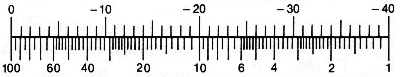percent

Conversion of distortion factor k as percent value %
to distortion attenuation
ak as damping in decibel (dB)

 Simply enter the value to the left or the right side. The calculator works in both directions of the ↔ sign. The distortion attenuation in dB has to begin with a minus sign.

 Distortion factor k (THD)  percent (%) ↔ Distortion attenuation a  dBk = THD0 dB ≡ 1 = 100 percent

Don't forget the minus sign, when entering the distortion attenuation in decibels.

The scales of VU meters show decibel values and percentage values.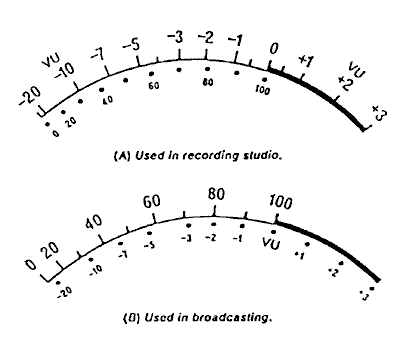Addition of harmonic distortion attenuation of multiple devices.

 Device 1 dB Device 2 dB Device 3 dB Device 4 dB Distortion sum dB